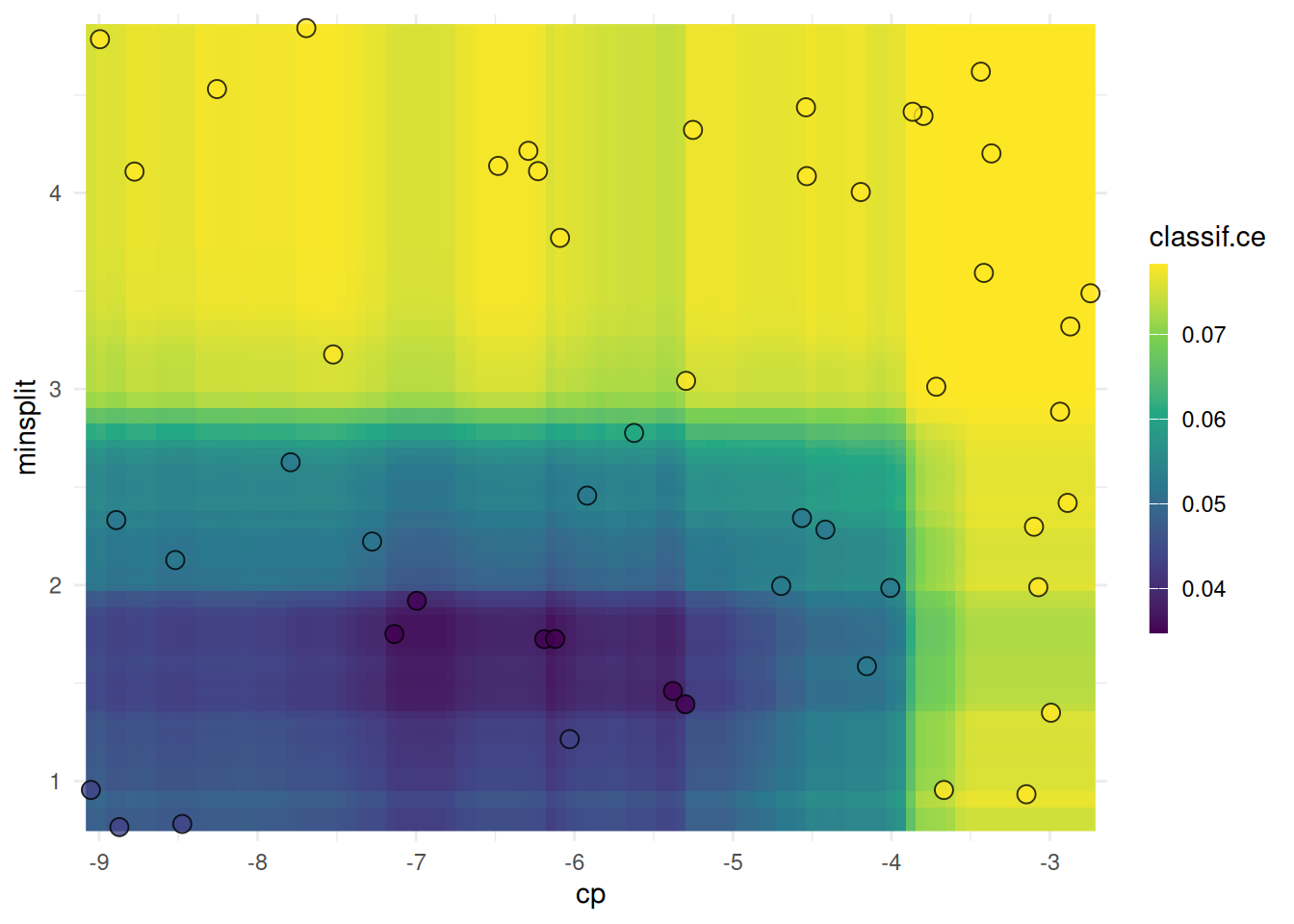# Tuners

Popular black-box optimization techniques are implemented in the bbotk package. The corresponding connectors to for tuning hyperparameters of learners or pipelines reside as `Tuner` objects in package mlr3tuning. Additionally, packages mlr3hyperband and mlr3mbo provide some modern and sophisticated approaches.

All tuners operator on box-constrained tuning spaces which have to be defined by the user. Some popular spaces from literature are readily available as tuning spaces.

## Example Usage

Tune the hyperparameters of a `classification tree` on the `Palmer Penguins` data set with `random search`.

``````library(mlr3verse)

# load learner and set search space
learner = lrn("classif.rpart",
cp = to_tune(1e-04, 1e-1, logscale = TRUE),
minsplit = to_tune(2, 128, logscale = TRUE)
)

# load tuner and set batch size
tuner = tnr("random_search", batch_size = 10)

# hyperparameter tuning on the palmer penguins data set
instance = tune(
method = tuner,
learner = learner,
resampling = rsmp("holdout"),
measure = msr("classif.ce"),
term_evals = 50
)

# best performing hyperparameter configuration
instance\$result``````
``````          cp minsplit learner_param_vals  x_domain classif.ce
1: -6.823753 1.435962          <list> <list> 0.03478261``````
``````# surface plot
autoplot(instance, type = "surface")````````````# fit final model on complete data set
learner\$param_set\$values = instance\$result_learner_param_vals
``````<LearnerClassifRpart:classif.rpart>: Classification Tree